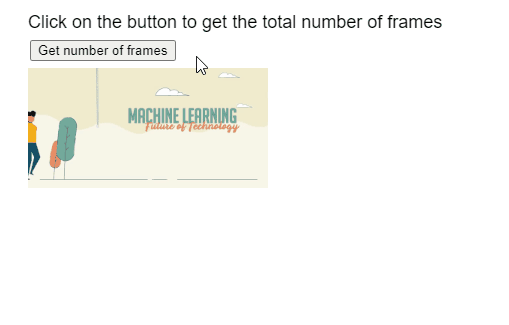# p5.js Image numFrames() Method

• Last Updated : 23 Sep, 2020

The numFrames() method of p5.Image in p5.js library is used to return the total number of frames of the GIF animation.

Syntax:

``` numFrames()
```

Parameters: This function accept does not accept any parameter.

Return Value: This method returns a number that represents the total number of frames of the GIF animation.

The following libraries are included in the “head” section of the HTML page while implementing the following examples.

<script src=”p5.Image.js”></script>
<script src=”p5.min.js”></script>

Example: The example below illustrates the numFrames() method in p5.js

## Javascript

 `function` `preload() { ` `    ``example_gif = ` `      ``loadImage(``"sample-gif.gif"``); ` `} ` ` `  `function` `setup() { ` `    ``createCanvas(500, 300); ` `    ``textSize(18); ` ` `  `    ``text(``"Click on the button to get "` `+ ` `         ``"the total number of frames"``, ` `         ``20, 20); ` ` `  `    ``frameBtn = ` `      ``createButton(``"Get number of frames"``); ` `    ``frameBtn.position(30, 40); ` `    ``frameBtn.mousePressed(getTotalFrames); ` `} ` ` `  `function` `draw() { ` ` `  `  ``// Draw the GIF on screen ` `  ``image(example_gif, 20, 60, 240, 120); ` `} ` ` `  `function` `getTotalFrames() ` `{ ` `  ``// Get the total number of frames ` `  ``let numberOfFrames = ` `      ``example_gif.numFrames(); ` ` `  `  ``text(``"Number of frames in the GIF is: "` `       ``+ numberOfFrames, 20, 200); ` `} `

Output:Online editor: https://editor.p5js.org/
Environment Setup: https://www.geeksforgeeks.org/p5-js-soundfile-object-installation-and-methods/
Reference: https://p5js.org/reference/#/p5.Image/numFrames

My Personal Notes arrow_drop_up
Recommended Articles
Page :